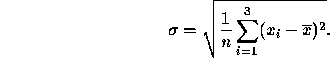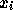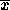# Midterm 1 Solution

CS 17

1. Short Answer Problems. Each of the following problems is worth 19 points.

1. (40 pts.) For each of the following expressions and the sub-expressions contained therein: determine the order of evaluation, the type, and the value.
1. ```int a = 6, b = 4, c = 5;
double x = 2.0;

a = ++b % c * x;
```

```++b, 5, int
++b % c, 0, int
++b % c * x, 0.0, double
a = ++b % c * x, 0, int
```

2. ```int a = 3; b = 4, c = 5, d = 6;
double x = 2.0;

d = a + b / x + c;
```

```b / x, 2.0, double
a + b / x, 5.0, double
a + b / x + c, 10.0, double
d = a + b / x + c, 10, int
```

3. ```int a = 3, b = 4, c = 5, d = 6, e = 7;

a = b + (c = d * e);
```

```d * e, 42, int
c = d * e, 42, int
b + (c = d * e), 46, int
a = b + (c = d * e), 46, int
```

4. ```double u = 2.0, w = 3.0, x = 5.0, y = 2.0, z = 3.0;

x += pow(y + z, 2.0) / w + u;
```

```y + z, 5.0, double
pow(y + z, 2.0), 25.0, double
pow(y + z, 2.0) / w, 8.333, double
pow(y + z, 2.0) / w + u, 10.333, double
x += pow(y + z, 2.0) / w + u, 15.333, double
```

2. (20 pts.) What is a general-purpose computer? A special-purpose computer? What is the most important difference between them?

A general-purpose computer is one that can run many different programs. A special-purpose computer is one which is dedicated to a single purpose.

The most important difference between them is that the general-purpose computer can be reprogrammed to perform many different tasks.

3. (20 pts.) Name the six aspects of the software life cycle.

1. Requirements specification.
2. Analysis.
3. Design.
4. Implementation.
5. Testing and verification.
6. Documentation.

4. (20 pts.) Name the seven requirements of an algorithm.

1. Input.
2. Output.
3. Un-ambiguousness.
4. Generality.
5. Correctness.
6. Finiteness.
7. Efficiency.

5. (15 pts.) Define the three structural elements of pseudocode.

• Sequence --- Statements execute sequentially, one after the other.
• Selection --- Choose between alternatives.
• Repetition --- Execute a set of statements repeatedly until a condition becomes false.

2. Programming Problems. Each of the following problems is worth 30 points. You are more likely to receive partial credit if you write your code so that I can understand what you've done.

1. Dr. Kelliher needs your help. He has a class with three students and needs you to write a C++ program to compute the average and standard deviation of exam scores. The input to the program is the three scores. The output should be the average and standard deviation. Here is the equation for the standard deviation:represents one exam score;is the average of the three exam scores. I will be glad to answer any questions regarding the interpretation of this equation.

Don't forget to include any necessary library files ( .h).

```#include <math.h>
#include <iostream.h>

int main()
{
double s1, s2, s3;   // scores
double ave, sum;

cout << "Enter score 1: ";
cin >> s1;
cout << "Enter score 2: ";
cin >> s2;
cout << "Enter score 3: ";
cin >> s3;

ave = (s1 + s2 + s3) / 3.0;

sum = pow(s1 - ave, 2.0) + pow(s2 - ave, 2.0) + pow(s3 - ave, 2.0);
sum /= 3.0;

cout << "\nAverage: " << ave;
cout << "\nStandard deviation: " << sqrt(sum) << endl;

return 0;
}
```

2. A standard science experiment is to drop a ball and see how high it bounces. Once the ``bounciness'' of the ball has been determined, the ratio gives a bounciness index. For example, if a ball dropped from a height of 10 feet bounces 6 feet hight, the index is 0.6 and the total distance traveled by the ball is 16 feet after one bounce. If the ball were to continue bouncing, the distance after two bounces would be 10 ft + 6 ft + 6 ft + 3.6 ft = 25.6 ft. Note that distance traveled for each successive bounce is the distance to the floor plus 0.6 of that distance as the ball comes back up.

Write pseudo-code that takes as input an initial ball height, a bounciness index, and a number of bounces. The output should be the total distance traveled by the ball.

```read the bounciness index

let distance = 0.0

while the number of bounces is greater than 0
let distance = distance + height
let height = height * bounciness index
let distance = distance + height
decrement by 1 the number of bounces
end_while

print the distance
```

Thomas P. Kelliher
Sat Mar 16 15:45:39 EST 1996
Tom Kelliher